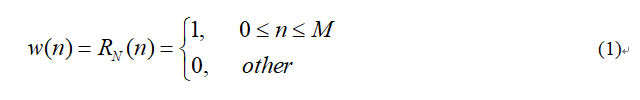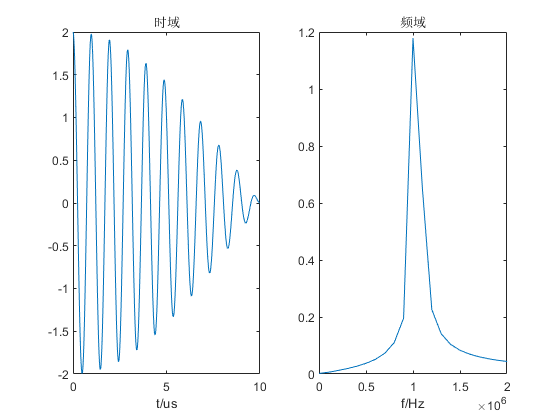• 频率分辨率
2021-05-20 22:15:36

1.fft补零：是频谱看起来更流畅（减小栅栏效应），但是不会改变实质。       补零只能说在原来的形状上插了更多的值，但是不会改变频率分辨率

在频率轴上的所能得到的最小频率间隔f0=fs/N=1/NTs=1/T,    由该公式可知，只有增加采样时长，才能增加。     补零并没有真正增加采样数据时长，故根本没有改变

更多相关内容
• MATLAB中使用FFT做频谱分析时频率分辨率问题-频率分辨率.rar MATLAB中使用FFT做频谱分析时频率分辨率问题 最近做FFT时，使用的采样频率和信号长度的取舍一直没有搞清楚，后来在论坛上发了一个贴子《总结一下使用...matlab
• 通俗的说频率分辨率就是你在一条直线（频率轴）上画竖线，相邻的两个竖线之间的间隔（你要分得清这两根线，不能黑乎乎的黏在一起）就是频率分辨率了，这种线画得越多频率分辨率自然就越高。 长度为T的一段信号因为...

定义
频率分辨率ΔfΔf 的定义是

其中 为采样周期， 为时域窗口长度（点数），为时域采样时间长度。
通俗的说频率分辨率就是你在一条直线（频率轴）上画竖线，相邻的两个竖线之间的间隔（你要分得清这两根线，不能黑乎乎的黏在一起）就是频率分辨率了，这种线画得越多频率分辨率自然就越高。

长度为T的一段信号因为是连续的，比较难想象，我们不妨将它转换为离散的，也就是说我们将它看成N个点的一段信号。这段信号的N越长，就意味着信号对应到频域上的点数越多，那 ΔfΔf自然是越小啦。
这里也有一个值得注意的地方，就是如果长度固定了，就是说采样率跟采样点数是成反比的，这就不存在说我们增加采样率或者是采样点数会让频率分辨率变好的情况，从公式就可以的出来的结论嘛~
时间域采样长度固定，采样间隔越小，点数越多，总时间T(窗函数长度）不变，其频率分辨率不变。频域的点数等于时域点数的一半，但是频域采样间隔与时域采样间隔成反比。

展开全文数字信号处理
• 本文主要对采样频率、采样点数、频率分辨率进行了解析说明，希望对你的学习有所帮助。
• 另外： 做DFT时，常常在有效数据后面补零达到对频谱做某种改善的目的，我们常常认为这是增加了N，从而使频率分辨率变好了，其实不是这样的，补零并没有增加有效数据的长度，仍然为T。但是补零其实有其他好处：1.使...

# 时间分辨率

时域图横坐标上的最小时间间隔。其大小代表着对模拟信号采样后得到的离散信号中点的密集程度。
数学公式：
T s = 1 F s {T_s} = \frac{1}{{{F_s}}}
数值越低，时间分辨率越高。
(图片来源https://zhuanlan.zhihu.com/p/130387384)点越密集，信号的时间分辨率越高。
所以，提高采样频率可以提高时域内的时间分辨率。

# 频率分辨率

频谱图横坐标上的最小频率间隔。
数学表达式：
d f = F s N = 1 N T s = 1 T df = \frac{{Fs}}{N} = \frac{1}{{N{T_s}}} = \frac{1}{T}

这时有两种情况。
1，分析一段完整的模拟信号。T确定，那频率分辨率就确定。这时如果增加N，必然减少Fs，那时间分辨率就低。
2，分析大量离散信号中的一部分。这时采样频率已知，那时间分辨率就确定。要想增加频率分辨率，就增加N。信号长度越长，频率分辨率越高。

另外：
做DFT时，常常在有效数据后面补零达到对频谱做某种改善的目的，我们常常认为这是增加了N，从而使频率分辨率变好了，其实不是这样的，补零并没有增加有效数据的长度，仍然为T。但是补零其实有其他好处：1.使数据N为2的整次幂，便于使用FFT。2.补零后，其实是对DFT结果做了插值，克服“栅栏”效应，使谱外观平滑化；“栅栏”效应形象理解为，就像站在栅栏旁边透过栅栏看外面风景，肯定有被栅栏挡住比较多风景，此时就可能漏掉较大频域分量，但是补零以后，相当于你站远了，改变了栅栏密度，风景就看的越来越清楚了。3.由于对时域数据的截短必然造成频谱泄露，因此在频谱中可能出现难以辨认的谱峰，补零在一定程度上能消除这种现象。

增加频率分辨率的方法：
信号的时间域分辨率和频率域分辨率

# 频率分辨率高低的直观表现

(图片来源https://zhuanlan.zhihu.com/p/130387384)如图：
原信号y=0.5sin(2pif1/fsn)+0.5sin(2pif2/fsn)。
图2、图3都是FFT后的频谱图，不同的是，图2频率分辨率较低，图3分辨率高。这张图可以很好的表明频率分辨率高低对信号处理的重要性。同时也说明处理离散信号时，N大小的选择尤为重要。

# STFT中的时间分辨率、频率分辨率

对信号进行短时傅里叶变换，窗的种类、窗的宽度和窗的滑移宽度都是一个问题。
窗太窄，窗内的信号太短，会导致频率分析不够精准，频率分辨率差。
窗太宽，时域上又不够精细，时间分辨率低。

展开全文• 为了提高GPS软件接收机信号捕获的载波频率分辨率，在频域并行频率空间搜索捕获方法中，利用加大分析数据长度可以提高频率分辨率的优点，结合频域并行码空间搜索捕获方法，构成了一种混合搜索方法。仿真结果表明本...
• 二、频率分辨率频率分辨率如何计算？怎样提高频率分辨率？三、频域采样栅栏效应如何缓解栅栏效应？四、MATLAB代码 一、时域加窗 频谱泄露 产生频谱泄露的原因是什么？ 如何抑制这一现象？ 二、频率分辨率 频率分辨率...

# 一、时域加窗

现实生活中的信号大部分是连续的，通过对连续的信号进行采样得到散时间信号，但是计算机所能处理的数据都是有限长的，因而我们可以对原始序列做加窗处理使其成为有限长序列。
以矩形窗为例，其时域表达式为：式(1)中， N = M + 1 N=M+1 ，为矩形窗的长度。

对无限长序列进行加窗处理，就是对序列在时域上乘以一个窗函数。
由卷积定理可以得到，时域的相乘等于频域的卷积。

设仿真信号的时域表达式为：
x ( t ) = A 0 ∗ c o s ( 2 π f 0 t ) + A 1 ∗ c o s ( 2 π f 1 t ) x(t)=A_{0}*cos(2πf_{0}t)+A_{1}*cos(2πf_{1}t)
x ( t ) x(t) 做傅里叶变换（FT）的频域表达式为：
X ( j Ω ) = A 0 π δ ( Ω + Ω 0 ) + A 0 π δ ( Ω − Ω 0 ) + A 1 π δ ( Ω + Ω 0 ) + A 1 π δ ( Ω − Ω 0 ) X(jΩ)=A_{0}πδ(Ω+Ω_{0})+A_{0}πδ(Ω-Ω_{0})+A_{1}πδ(Ω+Ω_{0})+A_{1}πδ(Ω-Ω_{0})
连续信号 x ( t ) x(t) 的波形及频谱如图1所示。连续信号 x ( t ) x(t) 经过采样后，得到的离散时间的表达式为：
x [ n ] = x ( t ) ∣ t = n T s x[n]=x(t)|_{t=nT_{s}}
离散序列 x [ n ] x[n] 做离散时间傅里叶变换（DTFT）的频域表达式为：
X ( e j w ) = 1 T s ∑ k = − ∞ ∞ X ( j w T s − j k 2 π T s ) X(e^{jw})=\frac{1}{T_{s}}\sum_{k=-∞}^{∞}X(j\frac{w}{T_{s}}-jk\frac{2π}{T_{s}})
离散序列 x [ n ] x[n] 的波形及频谱如图2所示。矩形窗函数 w [ n ] w[n] 做离散时间傅里叶变换（DTFT）的频域表达式为：
W ( e j w ) = e − j w ( N − 1 ) / 2 ∗ s i n ( w N / 2 ) s i n ( w / 2 ) W(e^{jw})=e^{-jw(N-1)/2} *\frac{sin(wN/2)}{sin(w/2)}

矩形窗函数 w [ n ] w[n] 的波形及频谱如图3所示。离散序列 x [ n ] x[n] 与窗函数 w [ n ] w[n] 的卷积为：
V ( e j w ) = 1 2 π ∫ − π π X ( e j θ ) W ( e j ( w − θ ) ) d θ = A 0 2 W ( e j ( w + w 0 ) ) + A 0 2 W ( e j ( w − w 0 ) ) + A 1 2 W ( e j ( w + w 0 ) ) + A 1 2 W ( e j ( w − w 0 ) ) V(e^{jw})=\frac{1}{2π}\int_{-π}^{π}X(e^{jθ})W(e^{j(w-θ)})dθ=\frac{A_{0}}{2}W(e^{j(w+w_{0})})+\frac{A_{0}}{2}W(e^{j(w-w_{0})})+\frac{A_{1}}{2}W(e^{j(w+w_{0})})+\frac{A_{1}}{2}W(e^{j(w-w_{0})})
截断后的离散序列 v [ n ] v[n] 的波形及频谱如图4所示。## 频谱泄露信号的频率成分包括1MHz和1.05MHz，1MHz对应的幅值为1，但是1.05MHz的幅值减小了，且在其他频率点上都有不小的幅值。这就是出现了频谱泄露的现象。

### 产生频谱泄露的原因是什么？

由于计算机只能处理有限长的数据，所以需要对采集的信号进行截断，相当于对原始信号做了加窗处理。对信号加窗就是对信号在时域上乘以一个窗函数，时域的乘积对应频域的卷积，而窗函数的频域包括主瓣和旁瓣，旁瓣造成了信号频谱的泄漏。频域泄漏不可避免，只能减小。

### 如何抑制这一现象？

可以取更长的数据点，与原始数据越接近越好，但缺点就是运算量加大；
可以选择窗谱的旁瓣能量较小的窗函数。
典型的窗函数中，矩形窗的频率分辨率最高，旁瓣泄露最大。

# 二、频率分辨率

## 频率分辨率如何计算？

为了便于理解什么是频率分辨率，可以将频率分辨率划分为两种类型，一种是波形频率分辨率（Waveform Frequency Resolution，简记为波形分辨率），也称为视觉频率分辨率，另一种则为FFT频率分辨率（简记为FFT分辨率）。

波形分辨率：在频谱图中，两个频率可以被分辨率的最小间隔，与原始信号的时间长度有关。
△ R w = 1 T △R_{w}=\frac{1}{T}
其中， T T 为原始数据的时长。

FFT分辨率：在频谱图中的数据点数，跟信号做FFT计算时的点数有关。
△ R f f t = F s N f f t △R_{fft}=\frac{F_{s}}{N_{fft}}
其中， F s F_{s} 为采样频率， N f f t N_{fft} 为信号做FFT计算时的点数。

## 怎样提高频率分辨率？

例如，有一个复合信号的时域表达式为 x ( t ) = c o s ( 2 π f 1 t ) + c o s ( 2 π f 2 t ) x(t)=cos(2πf_{1}t)+cos(2πf_{2}t)
其中 f 1 = 1 M H z f_{1}=1MHz f 2 = 1.05 M H z f_{2}=1.05MHz x ( t ) x(t) 的波形及频谱如图6所示。由上图中的频谱可以发现，在1MHz附近两个频率出现混叠，无法有效区分1MHz和1.05MHz，说明频率分辨率不够。

对时域数据进行补零，能否改变频率分辨率呢？例如在原始数据点后面再补充6000个数值为0的点，对信号本身数据没有影响，只是增加了参与FFT计算的数据点数，得到信号的波形及频谱如图7所示。通过对原始数据进行补零操作，在频谱图中的数据点更密集了。但是仍然无法区分1MHz和1.05MHz，由此证明信号的波形频率分辨率与参与FFT计算的数据点数 N f f t N_{fft} 无关，只与原始数据的时长 T T 有关。在时域上补零等价于频域上进行插值，由此增加了频率的点数，使得频谱曲线变得更光滑，即增加了FFT频率分辨率。

为了有效区分1MHz和1.05MHz，必须延长原始数据的时长以提高波形分辨率。以相同的采样频率对原始信号进行采样，采集7000个数据点。得到信号的波形及频谱如图8所示。有图8可见，1MHz和1.05MHz可以被区分开，但是也出现了频谱泄露现象。此时信号的波形分辨率为： △ R w = 1 70 u s ≈ 14 K H z △R_{w}=\frac{1}{70us}≈14KHz ，小于1MHz和1.05MHz之间的距离 50 K H z 50KHz

为了减小频谱泄露，对原始信号取更长的数据点，采集8000个数据点。得到信号的波形及频谱如图9所示。由图9可知，1MHz和1.05MHz对应的幅值均为1，且可以有效的区分开，此时信号的FFT分辨率为： △ R f f t = F s 8000 = 12.5 K H z △R_{fft}=\frac{F_{s}}{8000}=12.5KHz ，刚好是1MHz和1.05MHz的公约数，即 1 M H z = 12.5 K H z × 80 1MHz=12.5KHz×80 1.05 M H z = 12.5 K H z × 84 1.05MHz=12.5KHz×84
所以取合适长度的数据点可以减轻频谱泄露。

# 三、频域采样

周期序列的DFS的系数 X ( k ) X(k) x ( n ) x(n) 的一个周期的 Z Z 变换在单位圆的 N N 个均匀点上的抽样值相等，这就是频域采样。

频域采样定理：
N ≥ L N≥L ，即DFT计算的频域采样点数大于等于信号的长度时，频域采样不会造成时域混叠。
有限长序列 x ( n ) x(n) Z Z 变换为：
X ( Z ) = ∑ n = 0 N − 1 x ( n ) Z − n = ∑ n = 0 N − 1 [ 1 N ∑ k = 0 N − 1 X ( k ) W N − k n ] Z − n = 1 − Z − N N ∑ k = 0 N − 1 X ( k ) 1 − W N − k Z − 1 X(Z)=\sum_{n=0}^{N-1}x(n)Z^{-n}=\sum_{n=0}^{N-1}[\frac{1}{N}\sum_{k=0}^{N-1}X(k)W_{N}^{-kn}]Z^{-n}=\frac{1-Z^{-N}}{N}\sum_{k=0}^{N-1}\frac{X(k)}{1-W_{N}^{-k}Z^{-1}}
其中， W N − k n = e j 2 π k n N W_{N}^{-kn}=e^{j\frac{2πkn}{N}} W N − k = e j 2 π k N W_{N}^{-k}=e^{j\frac{2πk}{N}}

## 栅栏效应

在进行DFT计算时需要对信号的频域进行采样，由于采样间隔为 △ w = 2 π N △w=\frac{2π}{N} ，得到的频谱图都是由一根根离散的谱线组成，就像透过栅栏观看外景。## 如何缓解栅栏效应？

增加频域采样点数N（不改变时域数据的情况下，在时域数据末端添加一些零值点，使得谱线更密），可缩小谱线间距，减轻栅栏效应。

# 四、MATLAB代码

%% 1000个数据点的波形及频谱
clc;
clear;
close all;

Fs = 100e6;             % 采样频率
f1 = 1e6;f2 = 1.05e6;   % 信号的频率
T = 1/Fs;               % 采样周期
L0 = 1000;              % 信号长度
L = 1000;               % 数据长度
t0 = (0:L0-1)*T;        % 信号时间序列
t = (0:L-1)*T;          % 数据时间序列
x = cos(2*pi*f1*t0)+cos(2*pi*f2*t0);  % 原始信号

% FFT
[f1,A1] = PinPu(x,Fs);
figure(1)
subplot(1,2,1);plot(t*1e6,x);
xlabel('t/us');title('时域');
subplot(1,2,2);plot(f1,A1);
xlabel('f/Hz');title('频域');xlim([0 2e6]);

%% 出现了频谱泄露现象
clc;
clear;
close all;

Fs = 100e6;             % 采样频率
f1 = 1e6;f2 = 1.05e6;   % 信号的频率
T = 1/Fs;               % 采样周期
L0 = 7000;              % 信号长度
L = 7000;               % 数据长度
t0 = (0:L0-1)*T;        % 信号时间序列
t = (0:L-1)*T;          % 数据时间序列
x = cos(2*pi*f1*t0)+cos(2*pi*f2*t0);  % 原始信号
% FFT
[f1,A1] = PinPu(x,Fs);
figure(1)
subplot(1,2,1);plot(t*1e6,x);
xlabel('t/us');title('时域');
subplot(1,2,2);plot(f1,A1);
xlabel('f/Hz');title('频域');xlim([0 2e6]);

%% 8000个数据点的波形及频谱，提升了频率分辨率。
clc;
clear;
close all;

Fs = 100e6;             % 采样频率
f1 = 1e6;f2 = 1.05e6;   % 信号的频率
T = 1/Fs;               % 采样周期
L0 = 8000;              % 信号长度
L = 8000;               % 数据长度
t0 = (0:L0-1)*T;        % 信号时间序列
t = (0:L-1)*T;          % 数据时间序列
x = cos(2*pi*f1*t0)+cos(2*pi*f2*t0);  % 原始信号
% FFT
[f1,A1] = PinPu(x,Fs);
figure(1)
subplot(1,2,1);plot(t*1e6,x);
xlabel('t/us');title('时域');
subplot(1,2,2);plot(f1,A1);
xlabel('f/Hz');title('频域');xlim([0 2e6]);



# 参考文献

展开全文数字信号处理 频谱泄露
• 用Hilbert-Huang变换做时频分析的频率分辨率的实验研究，杨哂哂，李明，时频分析是分析时变谱的有力工具。其频率分辨率是值得研究的关键问题之一。本文作者对基于短时傅立叶变换、小波变换和Hilbert-Huang��
• 功率谱、频率分辨率、频谱泄漏与窗函数2008-06-01 23:49功率谱、频率分辨率、频谱泄漏与窗函数在分析和测定所采集的数据记录时，快速傅立叶变换(FFT)和功率谱是非常有用的工具。借助这些工具能够有效地采集时域信号...
• 这个概念容易理解，但是为了分辨系统信号的某一频率的值，即频率分辨率的概念让人有些迷惑。下面对于频率分辨率的概念进行详细的分析。 采样频率为fs，采样点为N，频率分辨率为fs/N。 而采样时间T等于N/fs。 所以...matlab
• 频率分辨率、补零问题 （1）当数据个数和FFT采用的数据个数均为32时，频率分辨率较低，但没有由于添零而导致的其他频率成分。 （2）由于在时间域内信号加零，致使振幅谱中出现很多其他成分，这是加零造成的。其振幅...
• ## 补零不能提高频率分辨率的原因

千次阅读 多人点赞 2020-06-16 16:45:43
在输出信号而言，相邻两个采样点的间隔为频率分辨率df=fs/N，其中fs为采样频率，N为输入信号的采样点数（N=fs*Ts），则频率分辨率df为： , 这也就是说，DFT的频率分辨率不仅与采样频率有关，也与信号的采样点数...
• 离散傅里叶变换(discrete Fourier transform) 傅里叶分析方法是信号分析的最基本方法，傅里叶变换是傅里叶分析的核心，通过它把信号从时间域变换到频率域，进而研究信号的频谱结构和变化规律。但是它的致命缺点是：...
• Matlab FFT变换细节(信号采样频率,FFT变换点数,频率分辨率)算法 matlab波形分辨率
• [FFT] matlab中关于FFT的使用(理解频率分辨率、补零问题)[FFT] matlab中关于FFT的使用(理解频率分辨率、补零问题)一.调用方法X=FFT(x)；X=FFT(x，N)；x=IFFT(X);x=IFFT(X,N)用MATLAB进行谱分析时注意：(1)函数FFT...
• 情况一：如果采样频率为fs，采样时间间隔为ts，采样点数为N，Nts就是采样前模拟信号的时间长度T，即采样时间为T（完成一组样本的采集所需要的时间），则频率分辨率： Δf=fs/N=1/Nts=1/T 所以信号长度越长，频率...数字信号处理
• 解释一：频率分辨率可以理解为在使用DFT时，在频率轴上的所能得到的最小频率间隔f0=fs/N=1/NTs=1/T,其中N为采样点数，fs为采样频率，Ts为采样间隔。所以NTs就是采样前模拟信号的时间长度T，所以信号长度越长，频率...
• 摘要：频率分辨率是频谱分析仪的一项重要技术指标，在介绍频谱分析仪原理的基础上，对频谱分析仪的分辨率带宽、频率选择性、本振残余调频和本振相位噪声参数进行了解析，从而使读者掌握各参数与频谱分析仪频率分辨率...
• 频率分辨率可以理解为在使用DFT时，在频率轴上的所能得到的最小频率间隔，即f0=fs/N=1/N∗Ts=1/Tf_0=f_s/N=1/N*T_s=1/Tf0​=fs​/N=1/N∗Ts​=1/T，其中NNN为采样点数，fsf_sfs​为采样频率，Ts=1/fsT_s=1/f_sTs​=1...
• 频率分辨率是频谱分析仪的一项重要技术指标，在介绍频谱分析仪原理的基础上，对频谱分析仪的分辨率带宽、频率选择性、本振残余调频和本振相位噪声参数进行了解析，从而使读者掌握各参数与频谱分析仪频率分辨率之间的...
• 频率分辨率的一些分析 最近被逼着写去除地震仪器响应的相关程序，不得不重新对本科学的数字信号处理的相关内容进行了学习，对于频率分辨率，做了一些分析．同时牵扯到了香农定律以及奈奎斯特频率（Nyquist ...数字信号处理 傅里叶变换
• 频率分辨率2. 时域补零的好处3. 时域补零实验图一：补零后长度为2048采样点图二：补零后长度为65536采样点（与原始音频长度接近）图三：补零长度到96000采样点4. 小结 1. 频率分辨率 频率分辨率是指对两个最近的...数字信号处理 语音识别
• matlab中关于FFT的使用(理解频率分辨率、补零问题)一.调用方法X=FFT(x)；X=FFT(x，N)；x=IFFT(X);x=IFFT(X,N)用MATLAB进行谱分析时注意：(1)函数FFT返回值的数据结构具有对称性。例：N=8;n=0:N-1;xn=[4 3 2 6 7 8 9 ...
• 在输出信号而言，相邻两个采样点的间隔为频率分辨率df=fs/N，其中fs为采样频率，N为输入信号的采样点数（N=fs*Ts），则频率分辨率df为： , 这也就是说，DFT的频率分辨率不仅与采样频率有关，也与信号的采样点数......